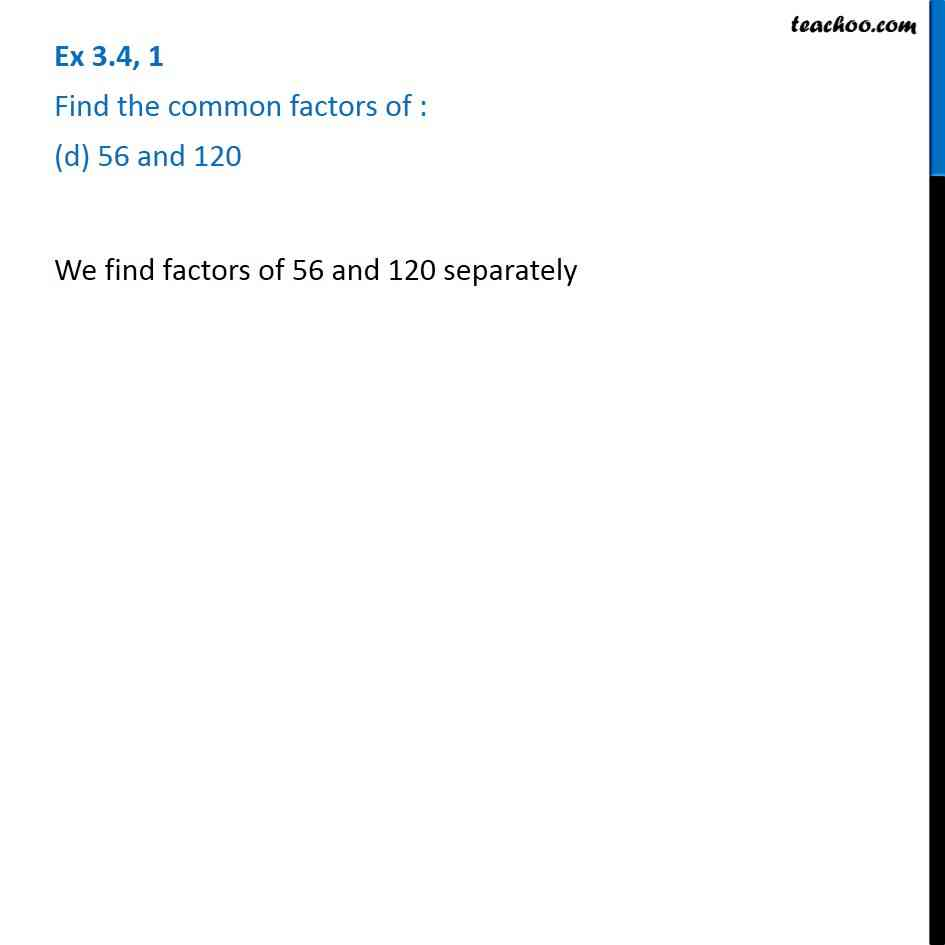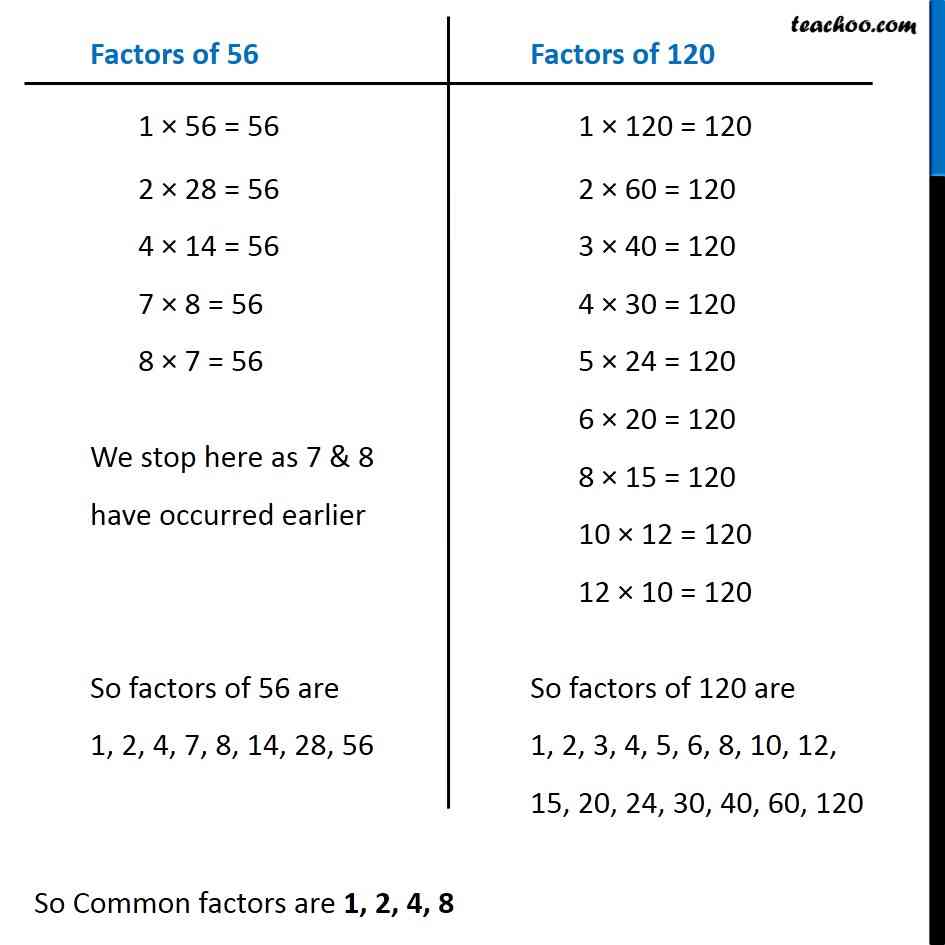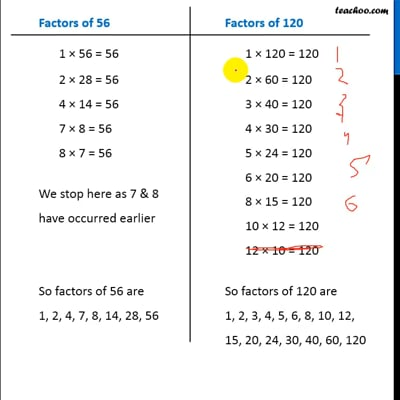Ex 3.4

Chapter 3 Class 6 Playing with Numbers
Serial order wiseThis video is only available for Teachoo black users

Introducing your new favourite teacher - Teachoo Black, at only ₹83 per month

### Transcript

Ex 3.4, 1 Find the common factors of : (d) 56 and 120 We find factors of 56 and 120 separately Factors of 56 1 × 56 = 56 2 × 28 = 56 4 × 14 = 56 7 × 8 = 56 8 × 7 = 56 We stop here as 7 & 8 have occurred earlier So factors of 56 are 1, 2, 4, 7, 8, 14, 28, 56 Factors of 120 1 × 120 = 120 2 × 60 = 120 3 × 40 = 120 4 × 30 = 120 5 × 24 = 120 6 × 20 = 120 8 × 15 = 120 10 × 12 = 120 12 × 10 = 120 So factors of 120 are 1, 2, 3, 4, 5, 6, 8, 10, 12, 15, 20, 24, 30, 40, 60, 120